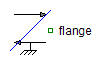Multiphase Variable Resistor - MapleSim Help

Multiphase Variable Resistor

Ideal linear electrical resistors with variable resistanceDescription The Multiphase Variable Resistor (or Variable Resistor) component contains $m$ single-phase variable resistor models, each connected between corresponding phases of ${\mathrm{plug}}_{p}$ and ${\mathrm{plug}}_{n}$, with the resistance controlled by the corresponding signal of an input vector.Connections

 Name Description Modelica ID ${\mathrm{plug}}_{p}$ Positive $m$-phase plug plug_p ${\mathrm{plug}}_{n}$ Negative $m$-phase plug plug_n $\mathrm{Heat Port}$ heatPort $R$ Real input $m$-vector; resistance in $\mathrm{\Omega }$ RParameters

 Name Default Units Description Modelica ID $m$ $3$ Number of phases m ${T}_{\mathrm{ref}}$ $\mathrm{fill}\left(300.15,m\right)$ $K$ Reference temperatures T_ref $\mathrm{\alpha }$ $\mathrm{zeros}\left(m\right)$ $\frac{1}{K}$ Temperature coefficients of resistances at reference temperatures alpha Use Heat Port $\mathrm{false}$ True (checked) means heat port is enabled useHeatPort $T$ ${T}_{\mathrm{ref}}$ $K$ Fixed device temperatures if Use Heat Port is false TModelica Standard Library The component described in this topic is from the Modelica Standard Library. To view the original documentation, which includes author and copyright information, click here.